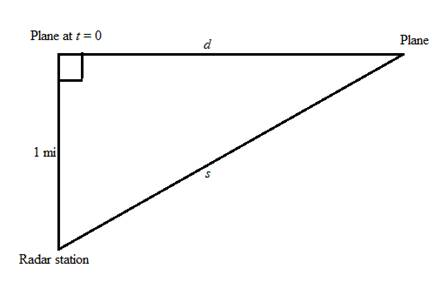# The function s that shows the distance between the plane and the radar station as a function of the horizontal distance d .### Precalculus: Mathematics for Calcu...

6th Edition
Stewart + 5 others
Publisher: Cengage Learning
ISBN: 9780840068071### Precalculus: Mathematics for Calcu...

6th Edition
Stewart + 5 others
Publisher: Cengage Learning
ISBN: 9780840068071

#### Solutions

Chapter 2.6, Problem 66E

(a)

To determine

## The function s that shows the distance between the plane and the radar station as a function of the horizontal distance d.

Expert Solution

The value of the function s is s(d)=1+d2 .

### Explanation of Solution

Given:

The speed of flying airplane is 350mi/h .

The airplane is flying at an altitude of 1 mile.

The plane passes directly above the radar station at time t=0 .

Formula used: Pythagoras Theorem for right angle triangle.

(hypotenuse)2=(perpendicular)2+(base)2

Calculation:

From the given information the airplane is flying at a speed of 350mi/h at an altitude 1mi and the plane passes directly above the radar station at time t=0 , therefore the given information forms a right angle triangle as shown below,Figure (1)

Since the function s that shows the distance between the plane and the radar station as a function of the horizontal distance d, therefore use the Pythagoras Theorem to find the function s.

s(d)=11+d2=1+d2

Thus, the value of the function s is s(d)=1+d2 .

(b)

To determine

### The function d that shows the distance at time t.

Expert Solution

The value of the function d is d(t)=350t .

### Explanation of Solution

Given:

The speed of flying airplane is 350mi/h .

Calculation:

Since the function d is the function that shows the distance at time t, therefore the function d is written as the product of speed of airplane and time t and the speed of airplane is 350mi/h .

d(t)=(350)(t)=350t

Thus, the value of the function d is d(t)=350t .

(c)

To determine

### The function s as a function of t by composition of functions.

Expert Solution

The function s as a function of t is expressed as (sd)(t)=1+122500t2 .

### Explanation of Solution

Given:

From part (a), the value of function s is given below,

s(d)=1+d2 (1)

From part (b), the value of function d is given below,

d(t)=350t (2)

Calculation:

The composite function sd is expressed as,

(sd)(t)=s(d(t))

From equation (2), substitute 350t for d(t) in above expression,

(sd)(t)=s(350t) (3)

Substitute 350t for d in equation (1), to find the value of s(350t) ,

s(350t)=1+(350t)2=1+122500t2

Substitute 1+122500t2 for s(350t) in equation (3), to find the value of sd ,

(sd)(t)=1+122500t2

The composite function (sd(t)) represents the function s as the function of time t.

Thus, the function s as a function of t is expressed as (sd)(t)=1+122500t2 .

### Have a homework question?

Subscribe to bartleby learn! Ask subject matter experts 30 homework questions each month. Plus, you’ll have access to millions of step-by-step textbook answers!